# 前言

Github项目--魔方

DirectX11 With Windows SDK完整目录

# 一个立方体绕魔方的旋转

struct Cube
{
// 获取当前立方体的世界矩阵
DirectX::XMMATRIX GetWorldMatrix() const;

RubikFaceColor faceColors;	// 六个面的颜色，索引0-5分别对应+X, -X, +Y, -Y, +Z, -Z面
DirectX::XMFLOAT3 pos;			// 旋转结束后中心所处位置
DirectX::XMFLOAT3 rotation;		// 仅允许存在单轴旋转，记录当前分别绕x轴, y轴, z轴旋转的弧度

};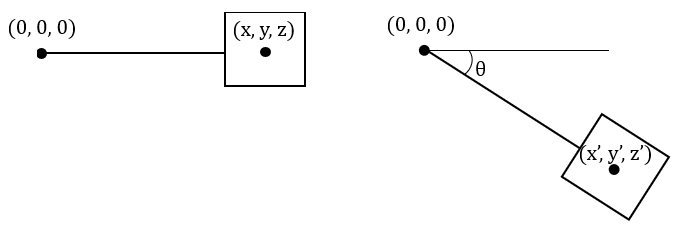$\mathbf{p'} = \mathbf{p} \times \mathbf{R_{z}(θ)}$

$\mathbf{v'} = \mathbf{v} \times \mathbf{R_{z}(θ)} \times \mathbf{T_{p'}}$

DirectX::XMMATRIX Cube::GetWorldMatrix() const
{
// rotation必然最多只有一个分量是非0，保证其只会绕其中一个轴进行旋转
XMMATRIX R = XMMatrixRotationRollPitchYaw(rotation.x, rotation.y, rotation.z);
posVec = XMVector3TransformCoord(posVec, R);
// 立方体转动后最终的位置
XMFLOAT3 finalPos;
XMStoreFloat3(&finalPos, posVec);

return XMMatrixRotationRollPitchYaw(rotation.x, rotation.y, rotation.z) *
XMMatrixTranslation(finalPos.x, finalPos.y, finalPos.z);
}


XMMatrixRotationRollPitchYaw函数是先按Z轴顺时针旋转，再按X轴顺时针旋转，最后按Y轴顺时针旋转。它实际上只会根据rotation来按其中一个轴旋转。

void Rubik::Update(float dt)
{
for (int i = 0; i < 3; ++i)
for (int k = 0; k < 3; ++k)
mCubes[i][k].rotation.y += XM_PI * dt;
}


void GameApp::UpdateScene(float dt)
{
mRubik.Update(dt);
}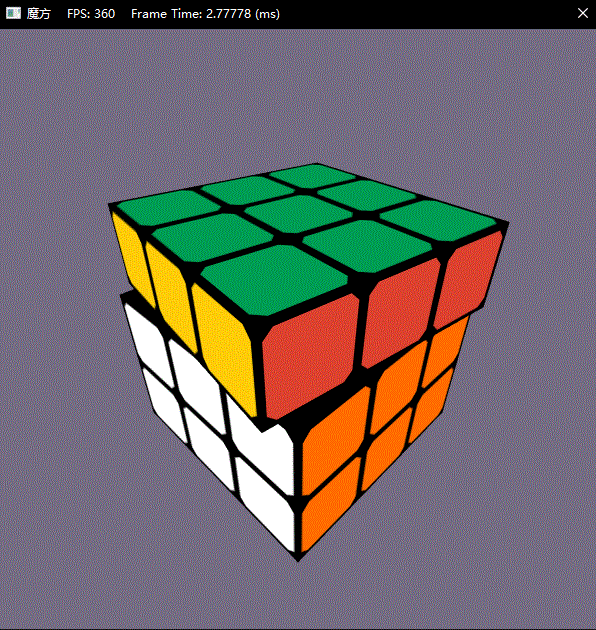# 魔方的旋转保护

class Rubik
{
public:
template<class T>
using ComPtr = Microsoft::WRL::ComPtr<T>;

Rubik();

// 初始化资源
void InitResources(ComPtr<ID3D11Device> device, ComPtr<ID3D11DeviceContext> deviceContext);
// 立即复原魔方
void Reset();
// 更新魔方状态
void Update(float dt);
// 绘制魔方
void Draw(ComPtr<ID3D11DeviceContext> deviceContext, BasicEffect& effect);
// 当前是否在进行动画中
bool IsLocked() const;

// pos的取值为0-2时，绕X轴旋转魔方指定层
// pos的取值为-1时，绕X轴旋转魔方pos为0和1的两层
// pos的取值为-2时，绕X轴旋转魔方pos为1和2的两层
// pos的取值为3时，绕X轴旋转整个魔方
void RotateX(int pos, float dTheta, bool isPressed = false);

// pos的取值为3时，绕Y轴旋转魔方指定层
// pos的取值为-1时，绕Y轴旋转魔方pos为0和1的两层
// pos的取值为-2时，绕Y轴旋转魔方pos为1和2的两层
// pos的取值为3时，绕Y轴旋转整个魔方
void RotateY(int pos, float dTheta, bool isPressed = false);

// pos的取值为0-2时，绕Z轴旋转魔方指定层
// pos的取值为-1时，绕Z轴旋转魔方pos为0和1的两层
// pos的取值为-2时，绕Z轴旋转魔方pos为1和2的两层
// pos的取值为3时，绕Z轴旋转整个魔方
void RotateZ(int pos, float dTheta, bool isPressed = false);

// 获取纹理数组

private:
// 绕X轴的预旋转
void PreRotateX(bool isKeyOp);
// 绕Y轴的预旋转
void PreRotateY(bool isKeyOp);
// 绕Z轴的预旋转
void PreRotateZ(bool isKeyOp);

// 获取需要与当前索引的值进行交换的索引，用于模拟旋转
// outArr1 { [X1][Y1] [X2][Y2] ... }
//              ||       ||
// outArr2 { [X1][Y1] [X2][Y2] ... }
void GetSwapIndexArray(int times, std::vector<DirectX::XMINT2>& outArr1,
std::vector<DirectX::XMINT2>& outArr2) const;

// 获取绕X轴旋转的情况下需要与目标索引块交换的面，用于模拟旋转
// cube[][Y][Z].face1 <--> cube[][Y][Z].face2
RubikFace GetTargetSwapFaceRotationX(RubikFace face, int times) const;
// 获取绕Y轴旋转的情况下需要与目标索引块交换的面，用于模拟旋转
// cube[X][][Z].face1 <--> cube[X][][Z].face2
RubikFace GetTargetSwapFaceRotationY(RubikFace face, int times) const;
// 获取绕Z轴旋转的情况下需要与目标索引块交换的面，用于模拟旋转
// cube[X][Y][].face1 <--> cube[X][Y][].face2
RubikFace GetTargetSwapFaceRotationZ(RubikFace face, int times) const;

private:
// 魔方 [X][Y][Z]
Cube mCubes;

// 当前是否鼠标正在拖动
bool mIsPressed;
// 当前是否有动画在播放
bool mIsLocked;
// 当前自动旋转的速度
float mRotationSpeed;

// 顶点缓冲区，包含6个面的24个顶点
// 索引0-3对应+X面
// 索引4-7对应-X面
// 索引8-11对应+Y面
// 索引12-15对应-Y面
// 索引16-19对应+Z面
// 索引20-23对应-Z面
ComPtr<ID3D11Buffer> mVertexBuffer;

// 索引缓冲区，仅6个索引
ComPtr<ID3D11Buffer> mIndexBuffer;

// 纹理数组，包含7张纹理
};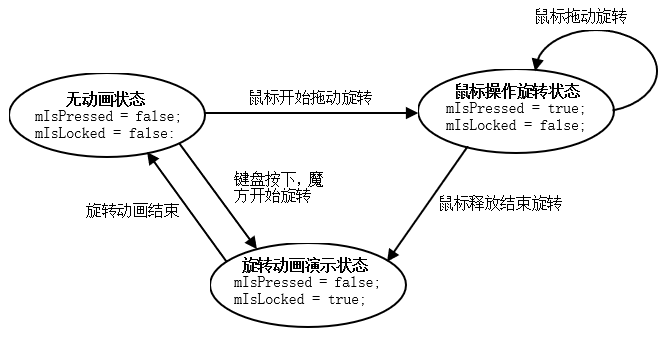mIsLockedtrue时，此时将会拒绝键盘或鼠标的响应，也就是说这个时候的旋转函数应该是不进行任何的操作。

// pos的取值为0-2时，绕X轴旋转魔方指定层
// pos的取值为-1时，绕X轴旋转魔方pos为0和1的两层
// pos的取值为-2时，绕X轴旋转魔方pos为1和2的两层
// pos的取值为3时，绕X轴旋转整个魔方
void RotateX(int pos, float dTheta, bool isPressed = false);


void Rubik::RotateX(int pos, float dTheta, bool isPressed)
{
if (!mIsLocked)
{
// 检验当前是否为键盘操作
// 可以认为仅当键盘操作时才会产生绝对值为pi/2的倍数(不包括0)的瞬时值
bool isKeyOp =  static_cast<int>(round(dTheta / XM_PIDIV2)) != 0 &&
(fabs(fmod(dTheta, XM_PIDIV2) < 1e-5f));
// 键盘输入和鼠标操作互斥，拒绝键盘的操作
if (mIsPressed && isKeyOp)
{
return;
}

mIsPressed = isPressed;

// ...

// 鼠标或键盘操作完成
if (!isPressed)
{

// 开始动画演示状态
mIsLocked = true;

// ...
}
}
}



# 魔方的旋转动画

void Rubik::RotateX(int pos, float dTheta, bool isPressed)
{
if (!mIsLocked)
{
// 检验当前是否为键盘操作
// 可以认为仅当键盘操作时才会产生绝对值为pi/2的倍数(不包括0)的瞬时值
bool isKeyOp =  static_cast<int>(round(dTheta / XM_PIDIV2)) != 0 &&
(fabs(fmod(dTheta, XM_PIDIV2) < 1e-5f));
// 键盘输入和鼠标操作互斥，拒绝键盘的操作
if (mIsPressed && isKeyOp)
{
return;
}

mIsPressed = isPressed;

// 更新旋转状态
for (int j = 0; j < 3; ++j)
for (int k = 0; k < 3; ++k)
{
switch (pos)
{
case 3: mCubes[j][k].rotation.x += dTheta;
case -2: mCubes[j][k].rotation.x += dTheta;
mCubes[j][k].rotation.x += dTheta;
break;
case -1: mCubes[j][k].rotation.x += dTheta;
mCubes[j][k].rotation.x += dTheta;
break;

default: mCubes[pos][j][k].rotation.x += dTheta;
}

}

// 鼠标或键盘操作完成
if (!isPressed)
{

// 开始动画演示状态
mIsLocked = true;

// 进行预旋转
PreRotateX(isKeyOp);
}
}
}



# 魔方的预旋转

## 操作完成后魔方按区间归位的问题

1. 把所有的终止条件都变为归位到0度，这样意味着只要rotation存在分量的值大于0，就需要让它逐渐减小到0；rotation存在分量的值小于0，就需要让它逐渐增加到0.
2. 我们可以在键盘按下，或者鼠标释放后动画即将开始的瞬间，立即对换所有准备旋转的立方体的表面，进行预旋转。这样正在执行的动画就只涉及普通的旋转操作了。

... ...
(-135°, 45°] -1
(-45°, 45°) 0
[45°, 135°) 1
... ...

$times = round(\frac{2θ}{\pi})$

// 由于此时被旋转面的所有方块旋转角度都是一样的，可以从中取一个来计算。
// 计算归位回[-pi/4, pi/4)区间需要顺时针旋转90度的次数
int times = static_cast<int>(round(mCubes[pos].rotation.x / XM_PIDIV2));
// 将归位次数映射到[0, 3]，以计算最小所需顺时针旋转90度的次数
int minTimes = (times % 4 + 4) % 4;


// 归位回[-pi/4, pi/4)的区间
mCubes[pos][j][k].rotation.x -= times * XM_PIDIV2;


// 顺时针旋转90度--->实际演算从-90度加到0度
// 逆时针旋转90度--->实际演算从90度减到0度
mCubes[pos][j][k].rotation.x *= -1.0f;


void Rubik::PreRotateX(bool isKeyOp)
{
for (int i = 0; i < 3; ++i)
{
// 当前层没有旋转则直接跳过
if (fabs(mCubes[i].rotation.x) < 10e-5f)
continue;
// 由于此时被旋转面的所有方块旋转角度都是一样的，可以从中取一个来计算。
// 计算归位回[-pi/4, pi/4)区间需要顺时针旋转90度的次数
int times = static_cast<int>(round(mCubes[i].rotation.x / XM_PIDIV2));
// 将归位次数映射到[0, 3]，以计算最小所需顺时针旋转90度的次数
int minTimes = (times % 4 + 4) % 4;

// 调整所有被旋转方块的初始角度
for (int j = 0; j < 3; ++j)
{
for (int k = 0; k < 3; ++k)
{
// 键盘按下后的变化
if (isKeyOp)
{
// 顺时针旋转90度--->实际演算从-90度加到0度
// 逆时针旋转90度--->实际演算从90度减到0度
mCubes[i][j][k].rotation.x *= -1.0f;
}
// 鼠标释放后的变化
else
{
// 归位回[-pi/4, pi/4)的区间
mCubes[i][j][k].rotation.x -= times * XM_PIDIV2;
}
}
}

// ...
}
}


## 实际的预旋转操作

1. 开启一个3x3的立方体临时数据，然后从源数据按旋转规则传递给临时数据，再复制回来。
2. 通过交换的方式完成就址旋转。

1. 怎么的交换顺序才能产生最终类似旋转的效果
2. 交换时两个立方体的六个面应该按怎样的规则来交换

### 交换实现旋转的原理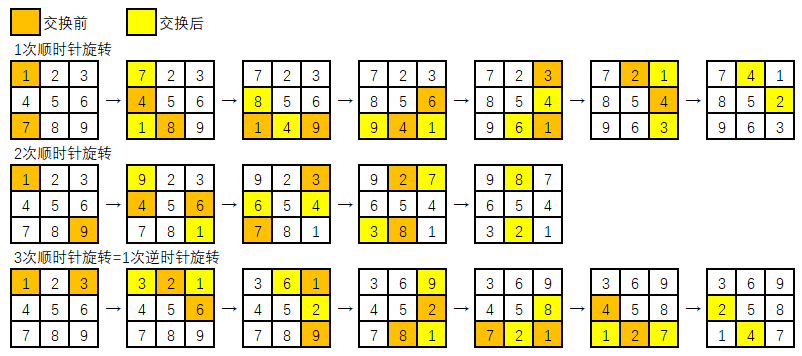void Rubik::GetSwapIndexArray(int minTimes, std::vector<DirectX::XMINT2>& outArr1, std::vector<DirectX::XMINT2>& outArr2) const
{
// 进行一次顺时针90度旋转相当逆时针交换6次(顶角和棱各3次)
// 1   2   4   2   4   2   4   1
//   *   ->  *   ->  *   ->  *
// 4   3   1   3   3   1   3   2
if (minTimes == 1)
{
outArr1 = { XMINT2(0, 0), XMINT2(0, 1), XMINT2(0, 2), XMINT2(1, 2), XMINT2(2, 2), XMINT2(2, 1) };
outArr2 = { XMINT2(0, 2), XMINT2(1, 2), XMINT2(2, 2), XMINT2(2, 1), XMINT2(2, 0), XMINT2(1, 0) };
}
// 进行一次顺时针90度旋转相当逆时针交换4次(顶角和棱各2次)
// 1   2   3   2   3   4
//   *   ->  *   ->  *
// 4   3   4   1   2   1
else if (minTimes == 2)
{
outArr1 = { XMINT2(0, 0), XMINT2(0, 1), XMINT2(0, 2), XMINT2(1, 2) };
outArr2 = { XMINT2(2, 2), XMINT2(2, 1), XMINT2(2, 0), XMINT2(1, 0) };
}
// 进行一次顺时针90度旋转相当逆时针交换6次(顶角和棱各3次)
// 1   2   4   2   4   2   4   1
//   *   ->  *   ->  *   ->  *
// 4   3   1   3   3   1   3   2
else if (minTimes == 3)
{
outArr1 = { XMINT2(0, 0), XMINT2(1, 0), XMINT2(2, 0), XMINT2(2, 1), XMINT2(2, 2), XMINT2(1, 2) };
outArr2 = { XMINT2(2, 0), XMINT2(2, 1), XMINT2(2, 2), XMINT2(1, 2), XMINT2(0, 2), XMINT2(0, 1) };
}
// 0次顺时针旋转不变，其余异常数值也不变
else
{
outArr1.clear();
outArr2.clear();
}

}


### 交换两个立方体表面时的规则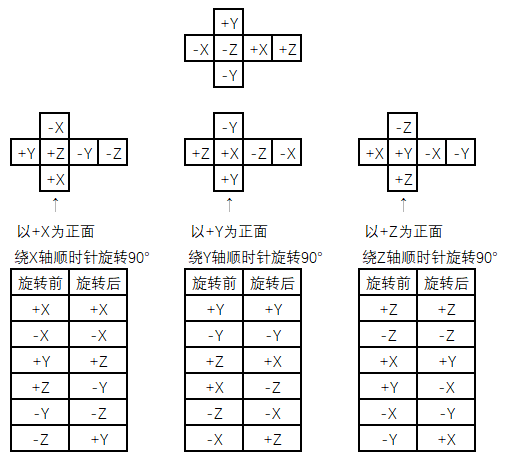RubikFace Rubik::GetTargetSwapFaceRotationX(RubikFace face, int times) const
{
if (face == RubikFace_PosX || face == RubikFace_NegX)
return face;
while (times--)
{
switch (face)
{
case RubikFace_PosY: face = RubikFace_NegZ; break;
case RubikFace_PosZ: face = RubikFace_PosY; break;
case RubikFace_NegY: face = RubikFace_PosZ; break;
case RubikFace_NegZ: face = RubikFace_NegY; break;
}
}
return face;
}

RubikFace Rubik::GetTargetSwapFaceRotationY(RubikFace face, int times) const
{
if (face == RubikFace_PosY || face == RubikFace_NegY)
return face;
while (times--)
{
switch (face)
{
case RubikFace_PosZ: face = RubikFace_NegX; break;
case RubikFace_PosX: face = RubikFace_PosZ; break;
case RubikFace_NegZ: face = RubikFace_PosX; break;
case RubikFace_NegX: face = RubikFace_NegZ; break;
}
}
return face;
}

RubikFace Rubik::GetTargetSwapFaceRotationZ(RubikFace face, int times) const
{
if (face == RubikFace_PosZ || face == RubikFace_NegZ)
return face;
while (times--)
{
switch (face)
{
case RubikFace_PosX: face = RubikFace_NegY; break;
case RubikFace_PosY: face = RubikFace_PosX; break;
case RubikFace_NegX: face = RubikFace_PosY; break;
case RubikFace_NegY: face = RubikFace_NegX; break;
}
}
return face;
}


void Rubik::PreRotateX(bool isKeyOp)
{
for (int i = 0; i < 3; ++i)
{
// 当前层没有旋转则直接跳过
if (fabs(mCubes[i].rotation.x) < 10e-5f)
continue;
// 由于此时被旋转面的所有方块旋转角度都是一样的，可以从中取一个来计算。
// 计算归位回[-pi/4, pi/4)区间需要顺时针旋转90度的次数
int times = static_cast<int>(round(mCubes[i].rotation.x / XM_PIDIV2));
// 将归位次数映射到[0, 3]，以计算最小所需顺时针旋转90度的次数
int minTimes = (times % 4 + 4) % 4;

// 调整所有被旋转方块的初始角度
for (int j = 0; j < 3; ++j)
{
for (int k = 0; k < 3; ++k)
{
// 键盘按下后的变化
if (isKeyOp)
{
// 顺时针旋转90度--->实际演算从-90度加到0度
// 逆时针旋转90度--->实际演算从90度减到0度
mCubes[i][j][k].rotation.x *= -1.0f;
}
// 鼠标释放后的变化
else
{
// 归位回[-pi/4, pi/4)的区间
mCubes[i][j][k].rotation.x -= times * XM_PIDIV2;
}
}
}

std::vector<XMINT2> indices1, indices2;
GetSwapIndexArray(minTimes, indices1, indices2);
size_t swapTimes = indices1.size();
for (size_t idx = 0; idx < swapTimes; ++idx)
{
// 对这两个立方体按规则进行面的交换
XMINT2 srcIndex = indices1[idx];
XMINT2 targetIndex = indices2[idx];
// 若为2次顺时针旋转，则只需4次对角调换
// 否则，需要6次邻角(棱)对换
for (int face = 0; face < 6; ++face)
{
std::swap(mCubes[i][srcIndex.x][srcIndex.y].faceColors[face],
mCubes[i][targetIndex.x][targetIndex.y].faceColors[
GetTargetSwapFaceRotationX(static_cast<RubikFace>(face), minTimes)]);
}
}
}
}


Rubik::RotateYRubik::RotateZ的实现这里忽略。

void Rubik::Update(float dt)
{
if (mIsLocked)
{
int finishCount = 0;
for (int i = 0; i < 3; ++i)
{
for (int j = 0; j < 3; ++j)
{
for (int k = 0; k < 3; ++k)
{
// 令x，y, z轴向旋转角度逐渐归0
// x轴
float dTheta = (signbit(mCubes[i][j][k].rotation.x) ? -1.0f : 1.0f) * dt * mRotationSpeed;
if (fabs(mCubes[i][j][k].rotation.x) < fabs(dTheta))
{
mCubes[i][j][k].rotation.x = 0.0f;
finishCount++;
}
else
{
mCubes[i][j][k].rotation.x -= dTheta;
}
// y轴
dTheta = (signbit(mCubes[i][j][k].rotation.y) ? -1.0f : 1.0f) * dt * mRotationSpeed;
if (fabs(mCubes[i][j][k].rotation.y) < fabs(dTheta))
{
mCubes[i][j][k].rotation.y = 0.0f;
finishCount++;
}
else
{
mCubes[i][j][k].rotation.y -= dTheta;
}
// z轴
dTheta = (signbit(mCubes[i][j][k].rotation.z) ? -1.0f : 1.0f) * dt * mRotationSpeed;
if (fabs(mCubes[i][j][k].rotation.z) < fabs(dTheta))
{
mCubes[i][j][k].rotation.z = 0.0f;
finishCount++;
}
else
{
mCubes[i][j][k].rotation.z -= dTheta;
}
}
}
}

// 所有方块都结束动画才能解锁
if (finishCount == 81)
mIsLocked = false;
}
}


void GameApp::UpdateScene(float dt)
{
// 反复旋转
static float theta = XM_PIDIV2;
if (!mRubik.IsLocked())
{
theta *= -1.0f;
}
// 就算摆出来也不会有问题(只有未上锁的帧才会生效该调用)
mRubik.RotateY(0, theta);
// 下面的也不会被调用
mRubik.RotateX(0, theta);
mRubik.RotateZ(0, theta);
// 更新魔方
mRubik.Update(dt);
}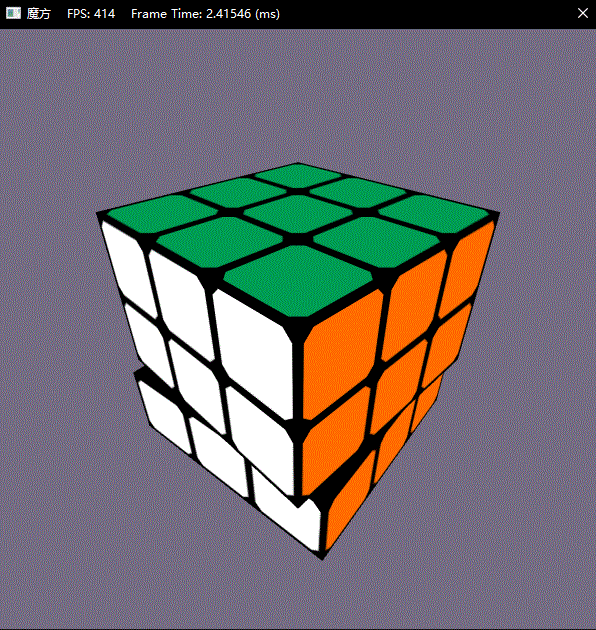Github项目--魔方

posted @ 2019-01-08 20:44  X_Jun  阅读(1583)  评论(7编辑  收藏  举报
levels of contents